Area of rectangles
Area of triangles
Compound area problems
Word problems
Area formulae
100

What is the area of the rectangle?Area = 4cm x 2cm
= 8cm2

100

What is the area of the below triangle?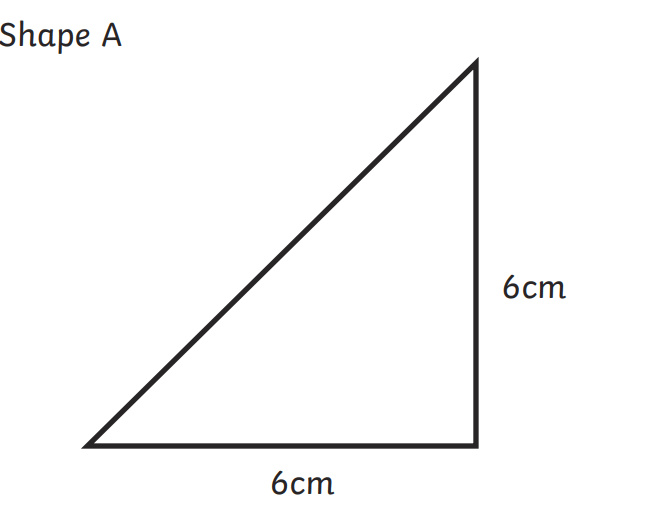Area = base x height / 2
= 6cm x 6cm /2
= 36cm2 /2
= 18cm2

100

What is the area of the below shape?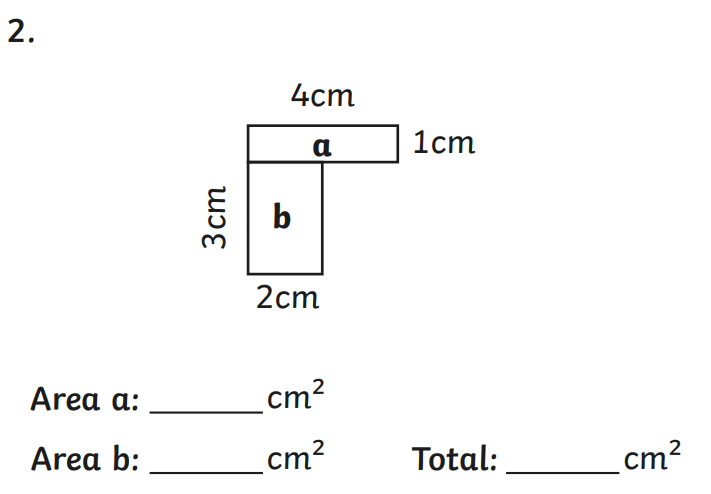Area a = 4cm2

Area b = 6cm2

Total area = a + b = 10cm2

100

A rectangle has a length of 3 metres and a width of 4 metres. What is the area of the rectangle?

3 metres x 4 metres = 12 metres2

3m x 4m = 12m2

100

How do you calculate the area of a rectangle?

Area of a rectangle = length x width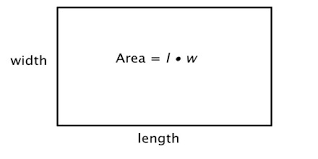200

What is the area of the rectangle below?Area = 11cm x 4cm
= 44cm2

200

What is the area of the triangle below?Area = base x height / 2
= 4cm x 6cm /2
= 24cm2 /2
= 12cm2

200

What is the area of the below shape?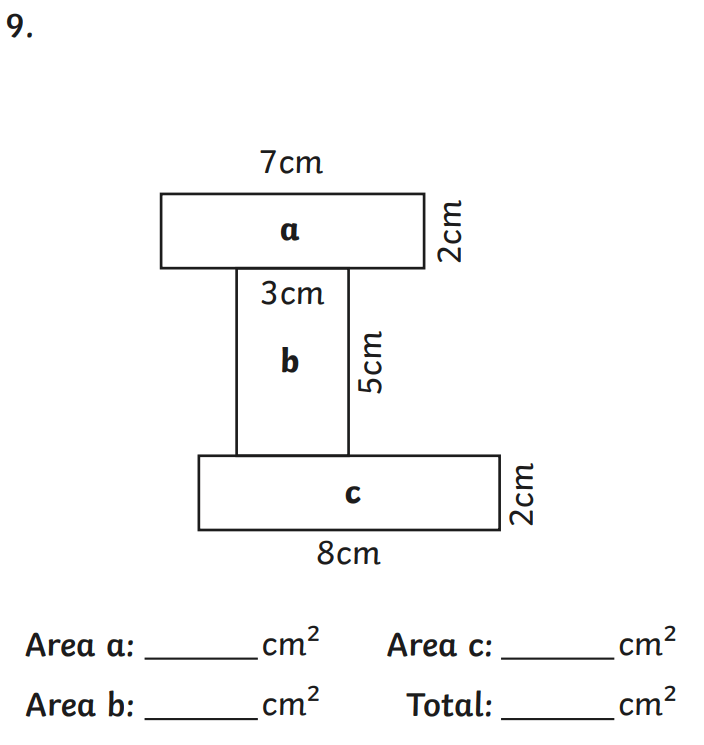Area a = 14cm2

Area b = 15cm2

Area c = 16cm2

Total area = a + b + c = 45cm2

200

A farmer has a square-shaped field where he keeps his cows. The length of one of the sides of the field is 30 metres. What is the area of the field?

30 metres x 30 metres = 900 metres2

30m x 30m = 900 m2

200

How do you calculate the area of a square?

Area of a square = side2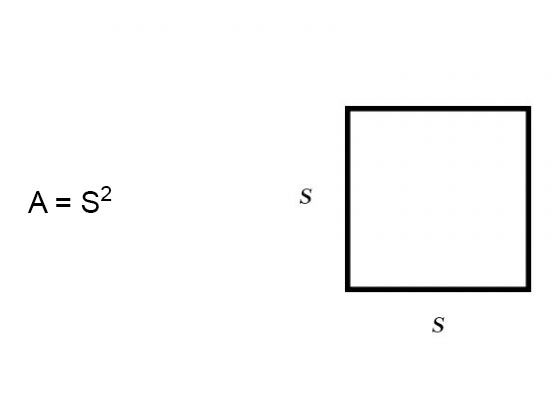300

What is the area of the rectangle below?Area = 20cm x 5cm
= 100cm2

300

What is the area of the triangle below?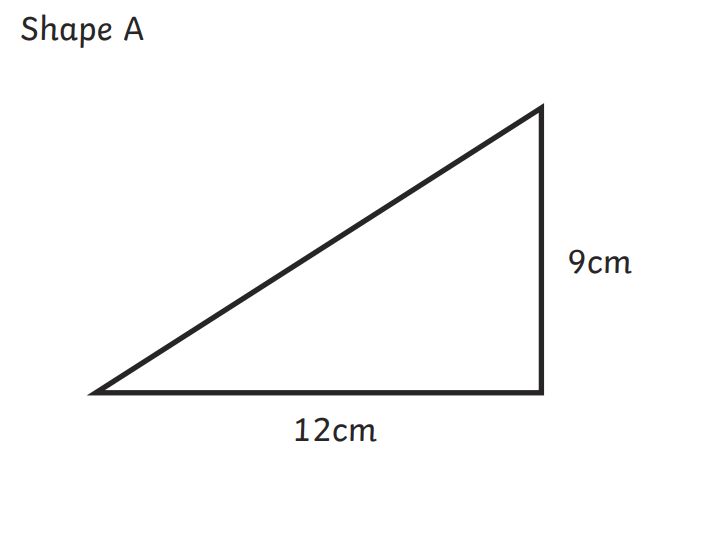Area = base x height / 2
= 9cm x 12cm /2
= 108cm2 /2
= 54cm2

300

What is the area of the below shape?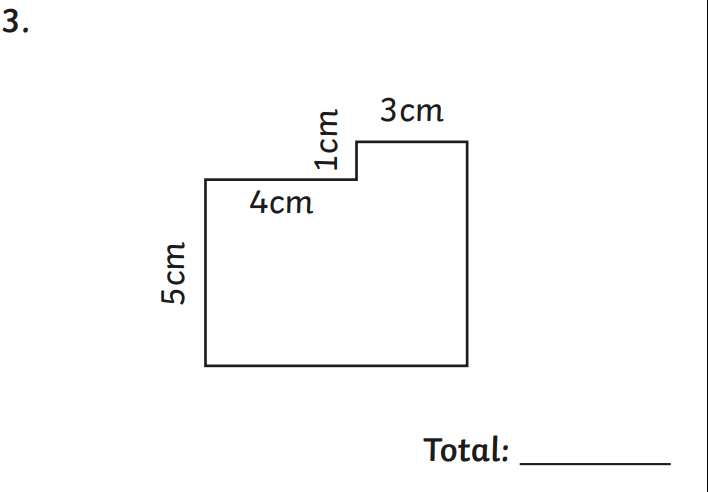Area a = 5cm x 4cm
= 20cm2

Area b = 6cm x 3cm
= 18cm2

Total area = a + b = 38cm2

300

A typical football field is about 120m long and 80m wide. What is the area of three football fields?

1 football field = 120 x 80
= 9600m2

3 football fields = 9600 x 3
= 28 800m2

300

How do you calculate an area like this?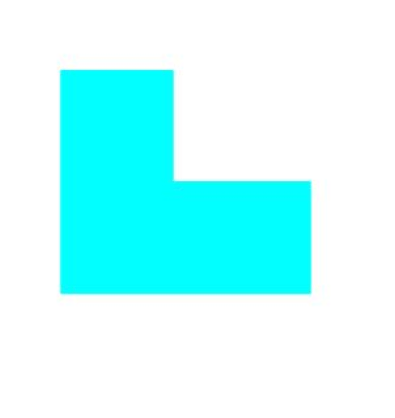Area of composite shape = divide the shape into two rectangles, find the areas of each and add them together.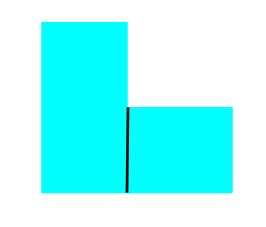400

What is the area of the rectangle below?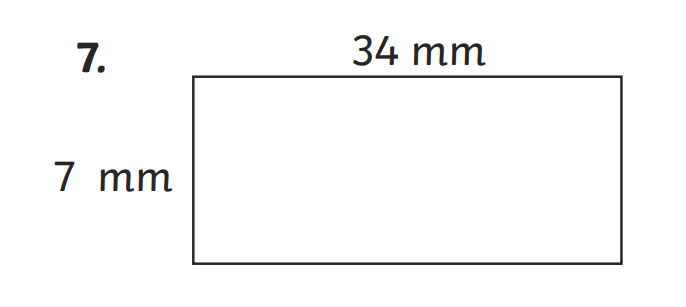Area = 34mm x 7mm
= 238mm2

400

What is the area of the triangle below?Area = base x height / 2
= 25cm x 60cm /2
= 1500cm2 /2
= 750cm2

400

What is the area of the below shape?Area a = 11m x 5m
= 55m2

Area b = 8m x 5m
= 40m2

Grey area = 8m x 3m
= 24m2

Total area = a + b - grey area
= 95 - 24
= 71m2

400

The area of a square is 64 cm2. What is the length of a side?

Area of a square = side x side

64cm2 = (number) x (number)

64cm= 8cm x 8cm

Length of a side = 8cm

400

How do you calculate the area of a triangle?

Area = base x height / 2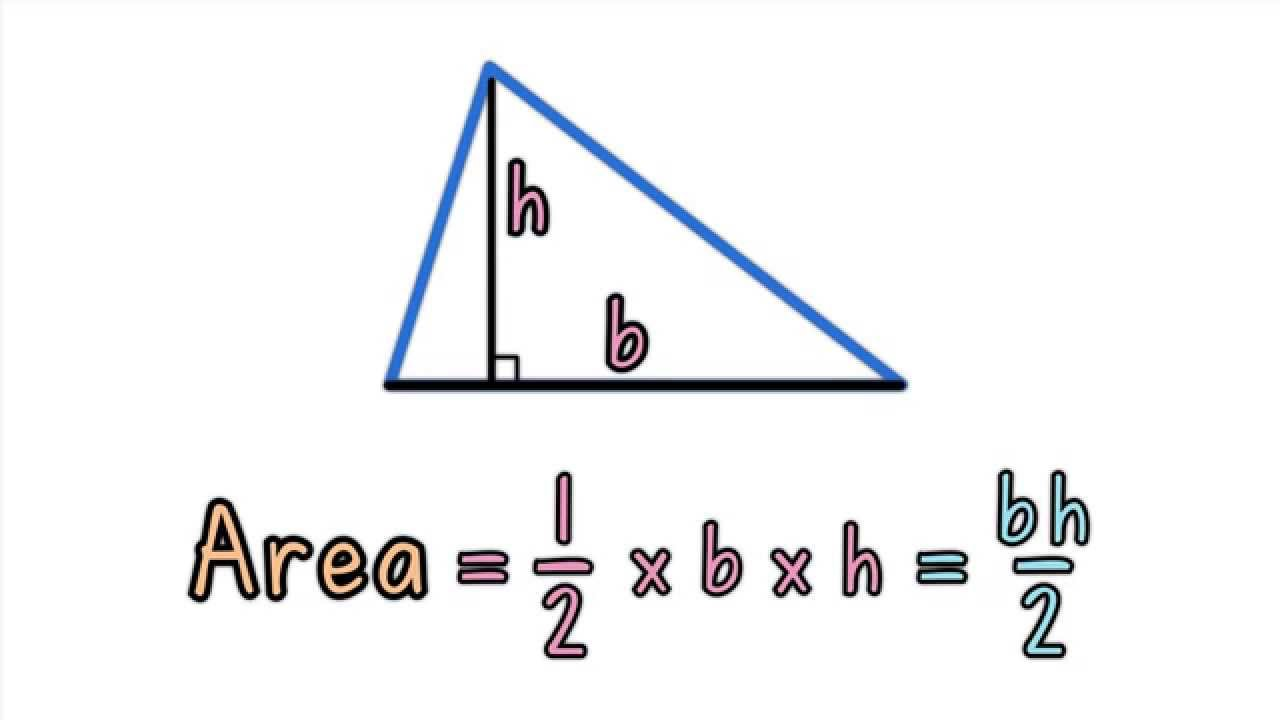500

What is the area of the rectangle below?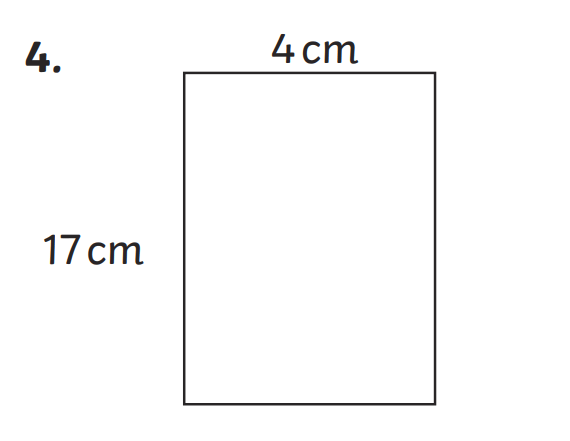Area = 4cm x 17cm
= 68cm2

500

What is the area of the triangle below?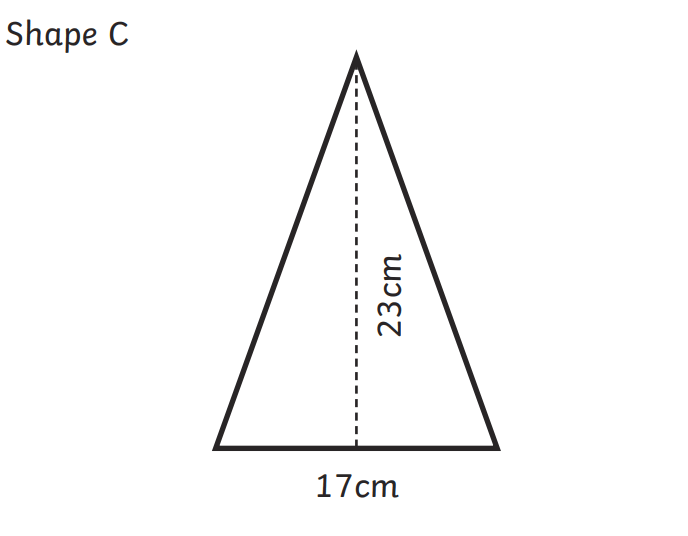Area = base x height / 2
= 23cm x 17cm /2
= 391cm2 /2
= 195.5cm2

500

What is the area of the below shape?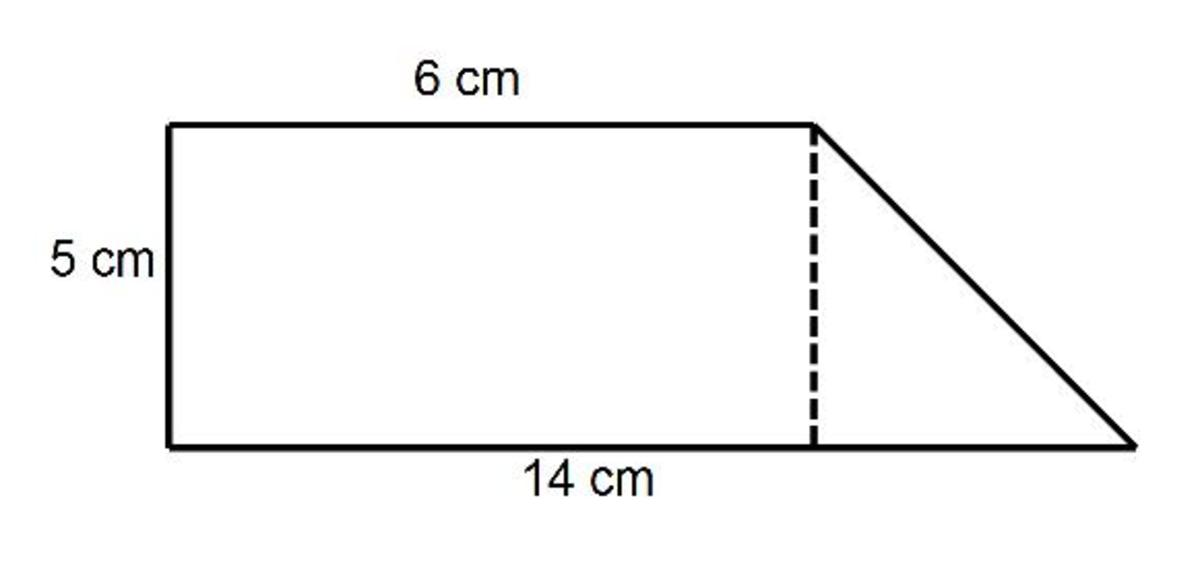Area a = 6cm x 5cm
= 30cm2

Base of triangle = 14-6
= 8cm

Area of triangle = base x height / 2
= 8 x 5 / 2
= 20cm2

Total area = 30cm2 + 20cm2
= 50cm2

500

A classroom has a length of 20 m and a width of 30 m. The principal decided that carpet tiles would look good in that class. If each tile has a length of 1m and a width of 2m, how many tiles would they need to cover the class?

Classroom = 20m x 30m
= 600m2

Tiles = 1m x 2m
= 2m2

Classroom / tiles = 600 / 2
= 300 tiles

500

How do you calculate an area like this?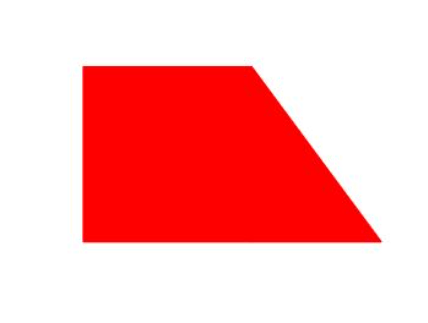Area of compound shape = divide the shape into a rectangle and a triangle, find the areas of each shape and then add them together.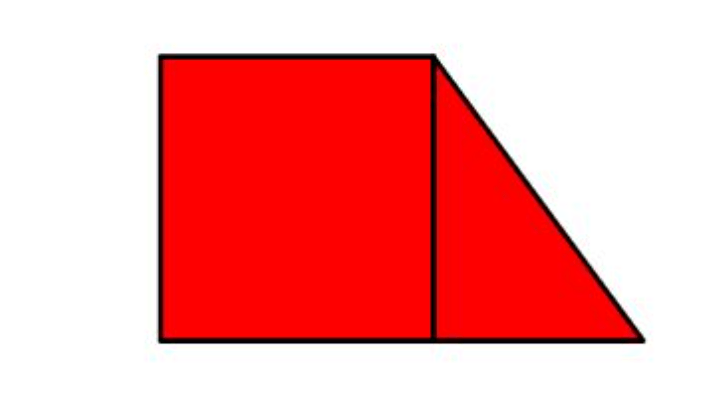Click to zoom